Home > Products > CAS Graphic Models > ClassPad II(fx-CP400) -Exploration Math

•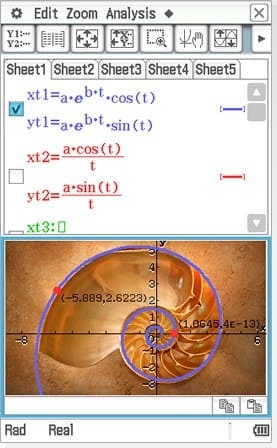(C) soupstock - Fotolia.com

•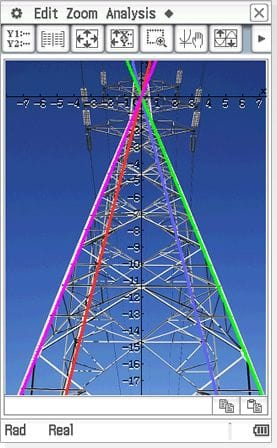(C) pn_photo - Fotolia.com

Real-Life Learning

Why we need to learn math?

Teaching mathematics in connection with real-life can be an effective method for keeping students interested. Moreover, we at CASIO have introduced our own special file format for images.

Explore the Mathematical Universe

Tap / Drag and Drop operation

•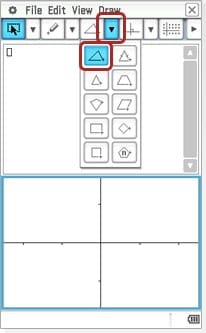•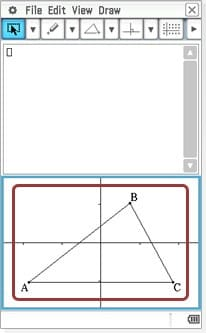Tap geometry area

Draw a Triangle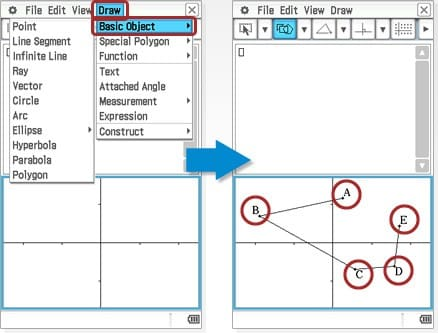Tap each vertex point

Draw a Polygon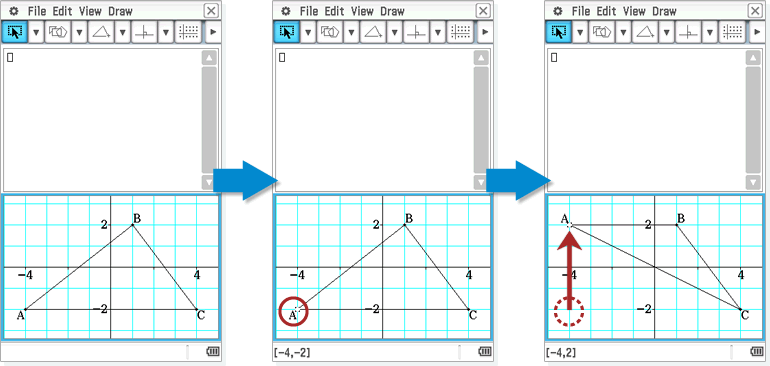Edit a Polygon

Drag and drop between windows to visualize mathematics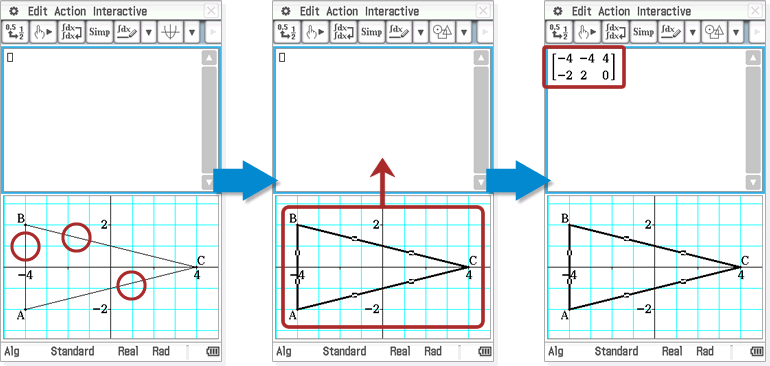• Tap each side to select a triangle

• Drag the triangle up to the Main section

• Formula for the triangle displayed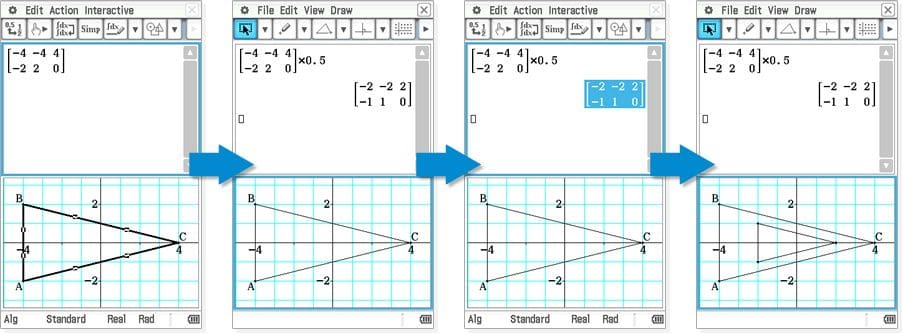• Triangle Algebraically

• Multiply by 0.5

Tap a matrix and drag to Geometry section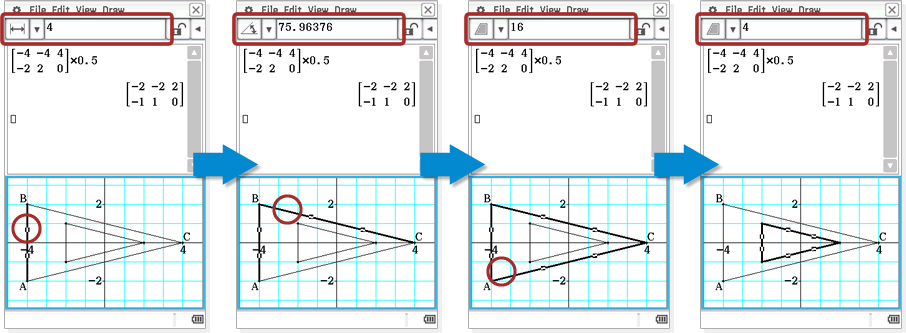• Tap AB
Length “AB”

• Tap BC
Angle “ABC”

• Tap AC
Area “ABC”

• Tap in any white space to deselect, then tap the smaller triangle